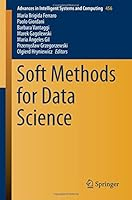# Soft Methods for Data Science0 Reviews
2016-08-30
535 pages

## Book Description

This proceedings volume is a collection of peer reviewed papers presented at the 8th International Conference on Soft Methods in Probability and (SMPS 2016) held in Rome (Italy).

The book is dedicated to Data science which aims at developing automated methods to analyze massive amounts of data and to extract knowledge from them. It shows how Data science employs various programming techniques and methods of data wrangling, data visualization, machine learning, probability and statistics. The soft methods proposed in this volume represent a collection of tools in these fields that can also be useful for data science.

Chapter 1. Mean Value and Variance of Fuzzy Numbers with Non-continuous Membership Functions
Chapter 2. On the of Radially Symmetric Trivariate Copulas
Chapter 3. Simulation of the Night Shift Solid Waste Collection System of Phuket Municipality
Chapter 4. Updating Context in the Equation: An Experimental Argument with Eye Tracking
Chapter 5. Black-Litterman Model with Multiple Experts' Linguistic Views
Chapter 6. Representing Lightweight Ontologies in a Product-Based Possibility Theory Framework
Chapter 7. Asymptotics of Predictive Distributions
Chapter 8. Independent k-Sample Equality Distribution Test Based on the Fuzzy Representation
Chapter 9. Agglomerative Fuzzy Clustering
Chapter 10. Bayesian Inference for a Finite Population Total Using Linked Data
Chapter 11. The Extension of Imprecise Probabilities Based on Generalized Credal Sets
Chapter 12. A Generalized SMART Fuzzy Disjunction of Volatility Indicators Applied to Option Pricing in a Binomial Model
Chapter 13. The Representation of Conglomerative Functionals
Chapter 14. The Likelihood Interpretation of Fuzzy Data
Chapter 15. Combining the Information of Multiple Ranker in Ranked Set Sampling with Fuzzy Set Approach
Chapter 16. A Savage-Like Representation Theorem for Preferences on Multi-acts
Chapter 17. On Some Functional Characterizations of (Fuzzy) Set-Valued Random
Chapter 18. Maximum Likelihood Under Incomplete Information: Toward a Comparison of Criteria
Chapter 19. The Use of Uncertainty to Choose Matching Variables in Statistical Matching
Chapter 20. Beyond Fuzzy, Possibilistic and Rough: An Investigation of Belief Functions in Clustering
Chapter 21. Small Area Estimation in the Presence of Linkage Errors
Chapter 22. A Test for Truncation Invariant Dependence
Chapter 23. Finite Mixture of Linear Regression Models: An Adaptive Constrained Approach to Maximum Likelihood Estimation
Chapter 24. A Multivariate Analysis of Tourists' Spending Behaviour
Chapter 25. Robust Fuzzy Clustering via Trimming and Constraints
Chapter 26. One-Factor Lévy-Frailty Copulas with Inhomogeneous Trigger Rates
Chapter 27. A Perceptron Classifier and Corresponding Probabilities
Chapter 28. Fuzzy Signals Fed to Gaussian Channels
Chapter 29. Fuzzy Clustering Through Robust Factor Analyzers
Chapter 30. Consensus-Based Clustering in Numerical Decision-Making
Chapter 31. Spatial Outlier Detection Using GAMs and Geographical Information
Chapter 32. Centering and Compound Conditionals Under Coherence
Chapter 33. Approximate Bayesian Methods for Multivariate and Conditional Copulae
Chapter 34. The Sign Test for Interval-Valued Data
Chapter 35. Probability Distributions Related to Fuzzy P-
Chapter 36. Probabilistic Semantics and Pragmatics for the Language of Uncertainty
Chapter 37. Dynamic Analysis of the Development of Scientific Communities in the Field of Soft
Chapter 38. Talk to Your Neighbour: A Belief Propagation Approach to Data Fusion
Chapter 39. The Qualitative Characteristics of Combining Evidence with Discounting
Chapter 40. Measuring the Dissimilarity Between the Distributions of Two Random Fuzzy Numbers
Chapter 41. An Empirical Analysis of the Coherence Between Fuzzy Rating Scale- and Likert Scale-Based Responses to Questionnaires
Chapter 42. Asymptotic Results for Sums of Independent Random Variables with Alternating Laws
Chapter 43. Dispersion Measures and Multidistances on mathbbRk
Chapter 44. Full Conglomerability, Continuity and Marginal Extension
Chapter 45. On Extreme Points of p-Boxes and Belief Functions
Chapter 46. Modelling the Dependence in Multivariate Longitudinal Data by Pair Copula Decomposition
Chapter 47. Predictability in Probabilistic Discrete Event Systems
Chapter 48. A Sandwich Theorem for Natural Extensions
Chapter 49. Envelopes of Joint Probabilities with Given Marginals Under Absolute Continuity or Equivalence Constraints
Chapter 50. Square of Opposition Under Coherence
Chapter 51. Testing of Coarsening Mechanisms: Coarsening at Random Versus Subgroup Independence
Chapter 52. Two-Sample Similarity Test for the Expected Value of Random Intervals
Chapter 53. Handling Uncertainty in Structural Equation Modeling
Chapter 54. Detecting Inconsistencies in Revision Problems
Chapter 55. Tukey's Biweight Loss Function for Fuzzy Set-Valued M-estimators of Location
Chapter 56. Technical Gestures Recognition by Set-Valued Hidden Markov Models with Prior Knowledge
Chapter 57. Time Series Modeling Based on Fuzzy Transform
Chapter 58. Back to ``Reasoning''
Chapter 59. Lexicographic Choice Functions Without Archimedeanicity
Chapter 60. Composition Operator for Credal Sets Reconsidered
Chapter 61. A Nonparametric Linearity Test for a Multiple Regression Model with Fuzzy Data
Chapter 62. Treat a Weak Dependence Among Causes and Lives in Insurance by Means of Fuzzy Sets
Chapter 63. A Portfolio Diversification Strategy via Tail Dependence Clustering
Chapter 64. An Upper Bound Estimation About the Sample Average of Interval-Valued Random Sets
Chapter 65. On Asymptotic Properties of the Multiple Fuzzy Least Squares Estimator

## Book Details

• Title: Soft Methods for Data Science
• Length: 535 pages
• Edition: 1st ed. 2017
• Language: English
• Publisher:
• Publication Date: 2016-08-30
• ISBN-10: 331942971X
• ISBN-13: 9783319429717